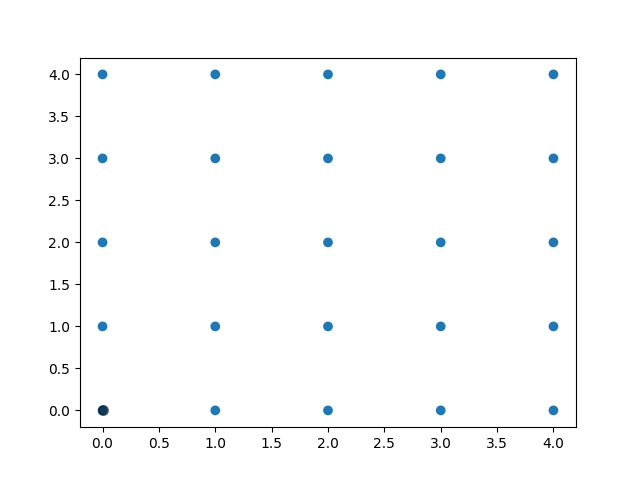# Polygon Selector Demo¶

Shows how one can select indices of a polygon interactively.Out:

Select points in the figure by enclosing them within a polygon.
Press the 'esc' key to start a new polygon.
Try holding the 'shift' key to move all of the vertices.
Try holding the 'ctrl' key to move a single vertex.

Selected points:
[]


import numpy as np

from matplotlib.widgets import PolygonSelector
from matplotlib.path import Path

class SelectFromCollection:
"""
Select indices from a matplotlib collection using PolygonSelector.

Selected indices are saved in the ind attribute. This tool fades out the
points that are not part of the selection (i.e., reduces their alpha
values). If your collection has alpha < 1, this tool will permanently
alter the alpha values.

Note that this tool selects collection objects based on their *origins*
(i.e., offsets).

Parameters
----------
ax : ~matplotlib.axes.Axes
Axes to interact with.
collection : matplotlib.collections.Collection subclass
Collection you want to select from.
alpha_other : 0 <= float <= 1
To highlight a selection, this tool sets all selected points to an
alpha value of 1 and non-selected points to *alpha_other*.
"""

def __init__(self, ax, collection, alpha_other=0.3):
self.canvas = ax.figure.canvas
self.collection = collection
self.alpha_other = alpha_other

self.xys = collection.get_offsets()
self.Npts = len(self.xys)

# Ensure that we have separate colors for each object
self.fc = collection.get_facecolors()
if len(self.fc) == 0:
raise ValueError('Collection must have a facecolor')
elif len(self.fc) == 1:
self.fc = np.tile(self.fc, (self.Npts, 1))

self.poly = PolygonSelector(ax, self.onselect)
self.ind = []

def onselect(self, verts):
path = Path(verts)
self.ind = np.nonzero(path.contains_points(self.xys))
self.fc[:, -1] = self.alpha_other
self.fc[self.ind, -1] = 1
self.collection.set_facecolors(self.fc)
self.canvas.draw_idle()

def disconnect(self):
self.poly.disconnect_events()
self.fc[:, -1] = 1
self.collection.set_facecolors(self.fc)
self.canvas.draw_idle()

if __name__ == '__main__':
import matplotlib.pyplot as plt

fig, ax = plt.subplots()
grid_size = 5
grid_x = np.tile(np.arange(grid_size), grid_size)
grid_y = np.repeat(np.arange(grid_size), grid_size)
pts = ax.scatter(grid_x, grid_y)

selector = SelectFromCollection(ax, pts)

print("Select points in the figure by enclosing them within a polygon.")
print("Press the 'esc' key to start a new polygon.")
print("Try holding the 'shift' key to move all of the vertices.")
print("Try holding the 'ctrl' key to move a single vertex.")

plt.show()

selector.disconnect()

# After figure is closed print the coordinates of the selected points
print('\nSelected points:')
print(selector.xys[selector.ind])


Keywords: matplotlib code example, codex, python plot, pyplot Gallery generated by Sphinx-Gallery